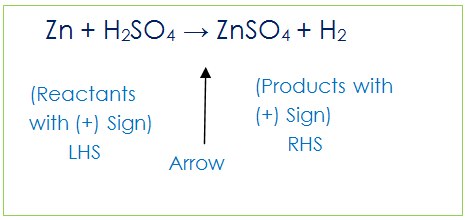Study Materials: CBSE Notes

In this section chapter 1. Chemical Reactions and Equations class 10 we will learn how to write a chemical equation and what is skeletal equation, we also learn about words equation and symbolic equation.

Notes ⇒ Class 10th ⇒ Science ⇒ 1. Chemical Reactions and Equations

# Notes 1. Chemical Reactions and Equations - Writing A chemical equation | Class 10 Science - Toppers Study

Topper Study classes prepares CBSE Notes on practical base problems and comes out with the best result that helps the students and teachers as well as tutors and so many ecademic coaching classes that they need in practical life. In this section chapter 1. Chemical Reactions and Equations class 10 we will learn how to write a chemical equation and what is skeletal equation, we also learn about words equation and symbolic equation.

## Notes 1. Chemical Reactions and Equations - Writing A chemical equation | Class 10 Science - Toppers Study

CBSE board students who preparing for class 10 ncert solutions maths and Science solved exercise chapter 1. Chemical Reactions and Equations available and this helps in upcoming exams 2023-2024.

### You can Find Science solution Class 10 Chapter 1. Chemical Reactions and Equations

• All Chapter review quick revision notes for chapter 1. Chemical Reactions and Equations Class 10
• NCERT Solutions And Textual questions Answers Class 10 Science
• Extra NCERT Book questions Answers Class 10 Science
• Importatnt key points with additional Assignment and questions bank solved.

Chapter 1 Science class 10

### Writing A chemical equation class 10 Science Chapter 1. Chemical Reactions and Equations

• Write The Chemical Equation
• What Is Chemical Equation
• Type Of Chemical Equation
• How To Write A Chemical Equation
• Examples Of Chemical Equation
• Words Equation Of Sodium Chloride
• What Is Reactant
• What Is Product
• What Is Words Chemical Equation
• What Is Symbolic Chemical Equation
• What Is Skeletal Equation

## Notes 1. Chemical Reactions and Equations - Writing A chemical equation | Class 10 Science - Toppers Study

### Chemical Equation:

when a magnesium ribbon is burnt in oxygen, it gets converted to magnesium oxide. This is the statement form of a chemical reaction but it can be write as a following manners

Magnesium + Oxygen → magnesium oxide

(Reactants)                      (Product)

To write the equation in such manner is called as word equation.

Another method to write chemical reaction;

Mg + O2 → MgO

This is symbolic form to write chemical equations.

Here we have known the types of chemical equations.

Theare are two types of chemical equations:

1. Words Equation

2. Symbolic Equation

### What is word chemical equation?

The chemical equation which is written by using elements name is called word equation

Example of words equation:

Sodium + chlorine → Sodium chloride

### What is symbolic chemical equation?

The chemical equation which is written by using elemets' symbol is called symbolic equation.

Example of symbolic equation:

Na + Cl → NaCl

There are two part of a chemical reaction

1. Reactants: The substances that undergo chemical change in the reaction are the reactants. Example from above equation. Mg and O are reactants.
2. Procducts: The new substance, formed during the reaction, is the product. Ex. MgO is product, which newer substance produced in reaction.

### What is reactant?

The substances that undergo chemical change in the reaction are the reactants. Example from above equation. Mg and O are reactants.

### What is product?

The new substance, formed during the reaction, is the product. Ex. MgO is product, which newer substance produced in reaction.

### Writing A chemical equation

A chemical equation represents a chemical reaction. So reactants are on the left hand side (LHS) of arrow and there is written (+) Sign between them. Similarly, Products are on the Right hand side (RHS) of arrow with a plus (+)  sign between them.

Consider this;### Skeletal Chemical Equation

Mg + O2 → MgO

Observe this equation, count and compare the number of atoms of each element on the LHS and RHS of the arrow. There is no equal numbers of atoms of each element on both sides. There is a little unbalance in number of atoms of Oxygen. There are 2 on left and 1 on the right of arrow. Unbalance chemical equation is called skeletal equation. hi